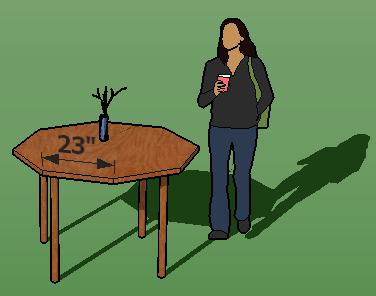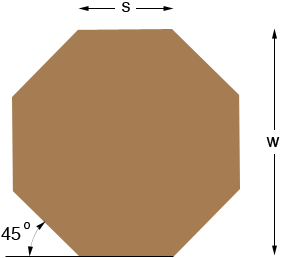SEARCH HOMEMath Central Quandaries & QueriesQuestion from Lorne, a parent: I am 77 years old and want to build a table top with each side measuring 23 inches. I believe the diameter would be 55.5 inches. Is this correct and what is the angle of the cuts I have to make? Thank you for your patience.Hi Lorne,In the answer to an earlier question Stephen showed that s = 0.4142 w where s and w are given in the diagram on the right above. I agree with your calculation, if s = 23 inches then w = 23 ÷ 0.4142 = 55.5 inches.

I'm not sure how you are going to construct the table so I don't know which angles you need. The exterior turning angles are 45o as in the diagram. Some other angles are given in the answer to an earlier question. Let us know if there is still something you need.

Penny and StephenMath Central is supported by the University of Regina and The Pacific Institute for the Mathematical Sciences.